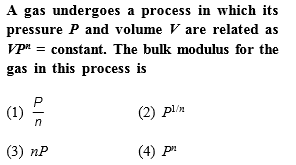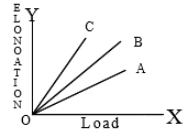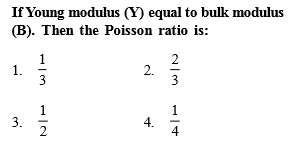[Only for Dropper and XII batch]

High Yielding Test Series + Question Bank - NEET 2020

Difficulty Level:

The young's modulus of a wire of length 'L' and radius 'r' is 'Y'. If length is reduced to L/2 and radius r/2 then young's modulus will be

1. Y/2

2. Y

3. 2Y

4. 4Y

High Yielding Test Series + Question Bank - NEET 2020

Difficulty Level:

Three wires A,B,C made of the same material and radius have different lengths. The graphs in the figure show the elongation-load variation. The longest wire is1. A

2. B

3. C

4. All

High Yielding Test Series + Question Bank - NEET 2020

Difficulty Level:

The breaking stress of a wire depends upon

1. material of the wire

2. length of the wire

4. shape of the cross section

High Yielding Test Series + Question Bank - NEET 2020

Difficulty Level:

The elastic energy stored in a wire of Young's modulus Y is -

1. $\mathrm{Y}×\frac{{\mathrm{strain}}^{2}}{\mathrm{volume}}$

2. $\mathrm{stress}×\mathrm{strain}×\mathrm{volume}$

3. $\frac{{\mathrm{strain}}^{2}×\mathrm{volume}}{2\mathrm{Y}}$

4. $\frac{1}{2}×stress×strain×vol$

High Yielding Test Series + Question Bank - NEET 2020

Difficulty Level:High Yielding Test Series + Question Bank - NEET 2020

Difficulty Level:

The bulk modulus of a spherical object is B. If it is subjected to uniform pressure p, the fractional decrease in radius is

(a)$\frac{P}{B}$

(b) $\frac{B}{3p}$

(c) $\frac{3p}{B}$

(d)$\frac{p}{3B}$

High Yielding Test Series + Question Bank - NEET 2020

Difficulty Level:

The Young's modulus of steel is twice that of brass. Two wires of same length and of same area of cross-section, one of steel and another of brass are suspended from the same roof. If we want the lower ends of the wires to be at the same level, then the weight added to the steel and brass wires must be in the ratio of

(a)1:2

(b)2:1

(c)4:1

(d)1:1

High Yielding Test Series + Question Bank - NEET 2020

Difficulty Level:

Copper of fixed volume V is drawn into wire of length l. When this wire is subjected to a constant force F, the extension produced in the wire is  Δl. Which of the following graphs is a straight line?

(a)Δl versues 1/l

(b)Δl versus l2

(c)Δl versus 1/l2

(d) Δl versus l

High Yielding Test Series + Question Bank - NEET 2020

Difficulty Level:

The following four wires are made of the same material. Which of then will have the largest extension when the same tension is applied?

(a) Length=50cm, diameter=0.5mm

(b) Length=100cm, diameter=1mm

(c) Length=200cm, diameter=2mm

(d) Length=300cm, diameter=3mm